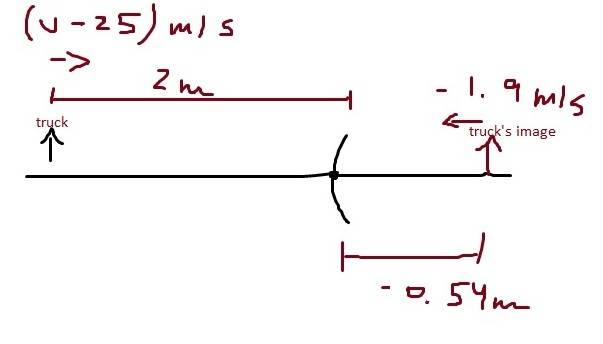# What is the speed?

## Homework Statement

You are in your car driving on a highway at 25 m/s when you glance in the passenger-side mirror (a convex mirror with radius of curvature 150 cm) and notice a truck approaching. If the image of the truck is approaching the vertex of the mirror at a speed of when the truck is 2.0 m from the mirror, what is the speed of the truck relative to the highway?

## The Attempt at a Solution

Assuming both the truck and the car speeds to be constant, the truck takes the same time interval as its image to reach the vertex of the mirror. So,

1 / f = 1 / s + 1 / s'
-1 / 0.75 m = 1 / 2 m + 1 / s'
s' = -0.54 m
t = -0.54 m / -1.9 m /s
[ (V - 25) m / s ] t = 2 m
but solving this for V I didnt find the correct value: 51 m / s. Maybe the speed is'nt constant?

My sketch:haruspex
Homework Helper
Gold Member
2020 Award
The speed of the image will not be constant. You can see this by differentiating the 1/f formula.

I dont know how to do thisharuspex
Homework Helper
Gold Member
2020 Award
I dont know how to do thisThe derivative of s with respect to time, ds/dt, is the velocity, v. What is the derivative of 1/s? Use the chain rule.

hmmmm let me try...

1 / f = 1 / V dt + 1 / V' dt
1 / f = 1 / dt (1 / V + 1 / V')

but how can I solve this derivative? would I need to express f as function of t?

Last edited:
haruspex
Homework Helper
Gold Member
2020 Award
hmmmm let me try...

1 / f = 1 / V dt + 1 / V' dt
1 / f = 1 / dt (1 / V + 1 / V')

but how can I solve this derivative? would I need to express f as function of t?
f is a constant. What is the derivative of a constant?
The chain rule says d/dt(1/s)= (ds/dt)(d/ds(1/s)). What is d/ds(1/s)?

ohh yah

(d / dt) 1 / f = 0
0 = - [(V - 25 m / s) / s²] - V' / s'²
V ≅ 51 m / s

Thanksbut why the image speed is not constant?

Last edited:
haruspex
Homework Helper
Gold Member
2020 Award
ohh yah

(d / dt) 1 / f = 0
0 = - [(V - 25 m / s) / s²] - V' / s'²
V ≅ 51 m / s

Thanksbut why the image speed is not constant?
Because the relationship between the two distances is nonlinear.